# Lakhmir Singh Solutions Class 9 Physics Chapter 2 Force and Laws of Motion

Force is defined as the push or pull exerted on an object which produces acceleration in an object. Newton is the SI unit of force. Following are the three changes that can be observed on a body when force is applied:

• Change in the direction of motion of the body
• Change in the speed of the body
• Change in the shape of the body

Force can be either balanced or unbalanced. Force is said to be balanced when they cancel out each other such that the resultant force is zero. When the force acting on a body moves the body in the direction of force which is greater than the other, it is known as unbalanced force.

Laws of Motion

There are three laws of motion and they are:

• First law of motion: According to the first law of motion, an object remains to be in a state of rest or in a state of uniform motion unless acted upon by a force. This law is also known as the law of inertia.
• Second law of motion: According to the second law of motion, the change in momentum of an object is proportional to the applied force and is in the same direction as the force.
• Third law of motion: According to the third law of motion, there is an equal and opposite reaction for every action and they act on two different objects.

## Download PDF Of Lakhmir Singh Physics Class 9 Solutions For Chapter 2 Force and Laws of Motion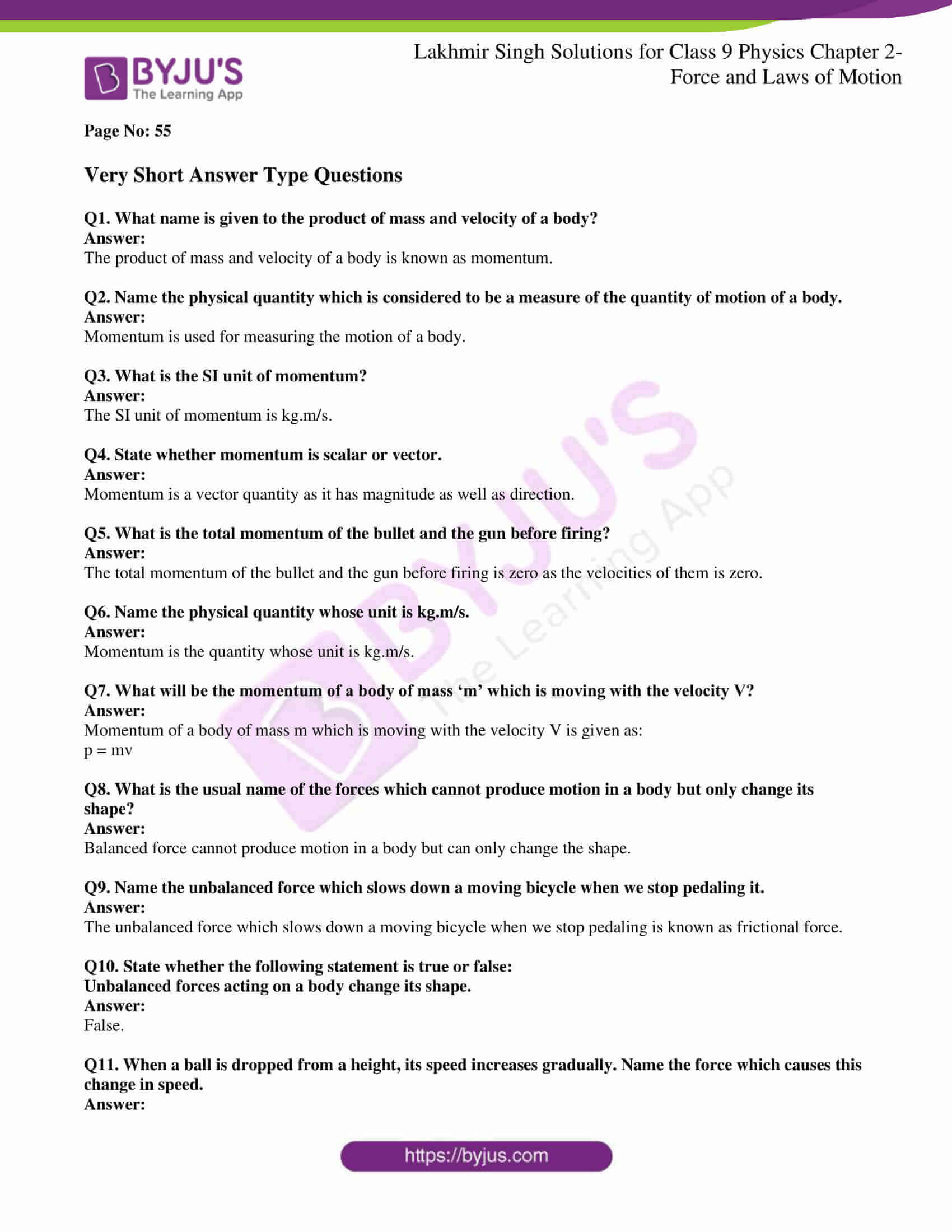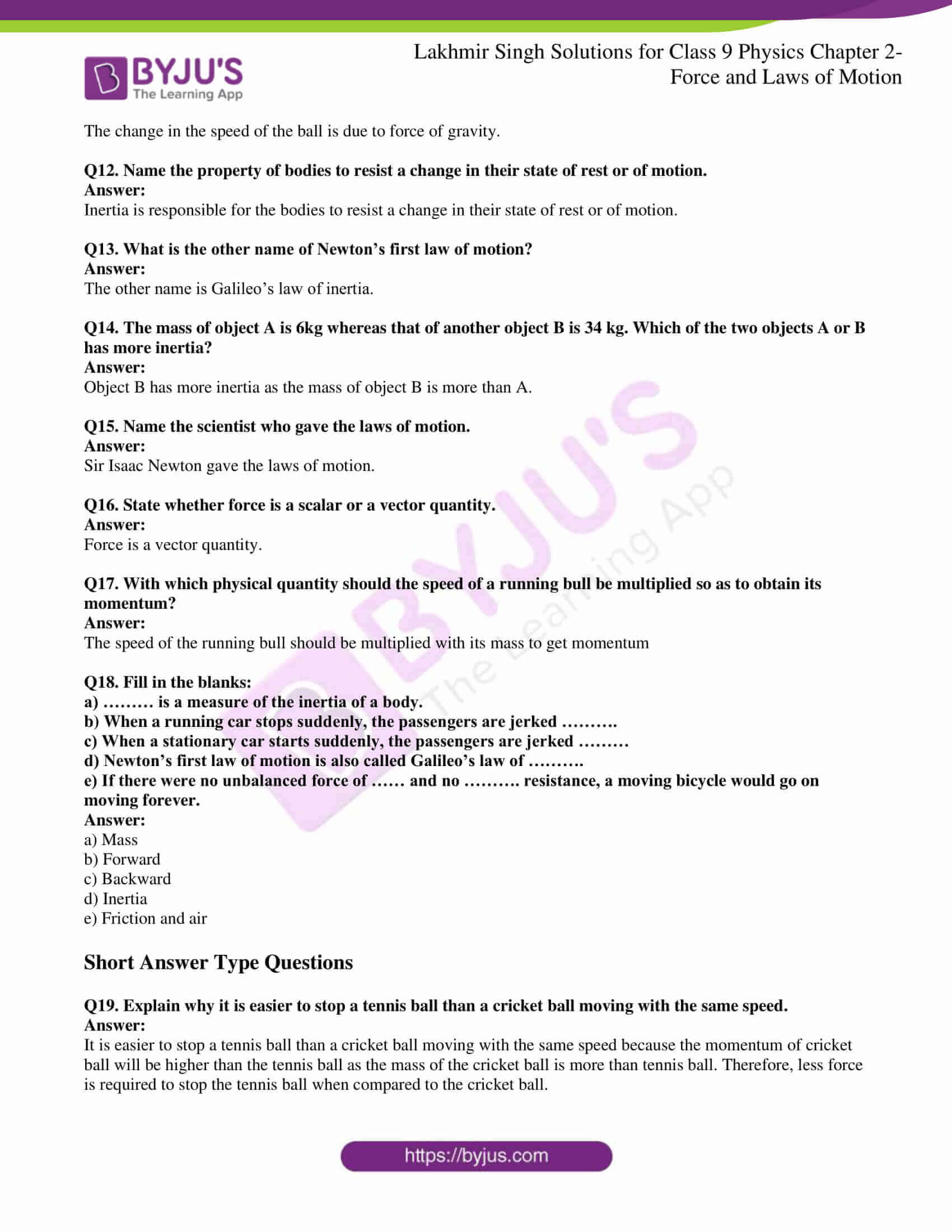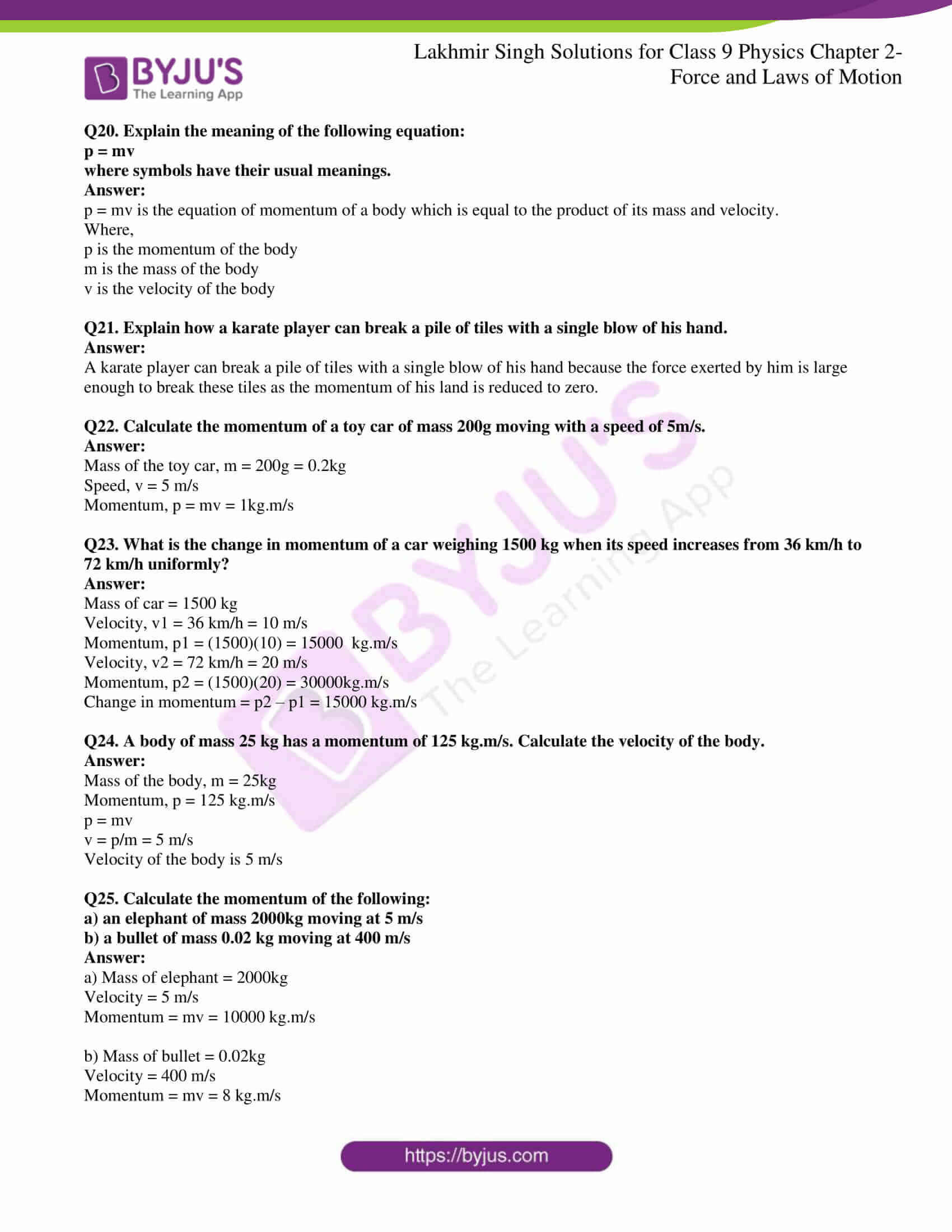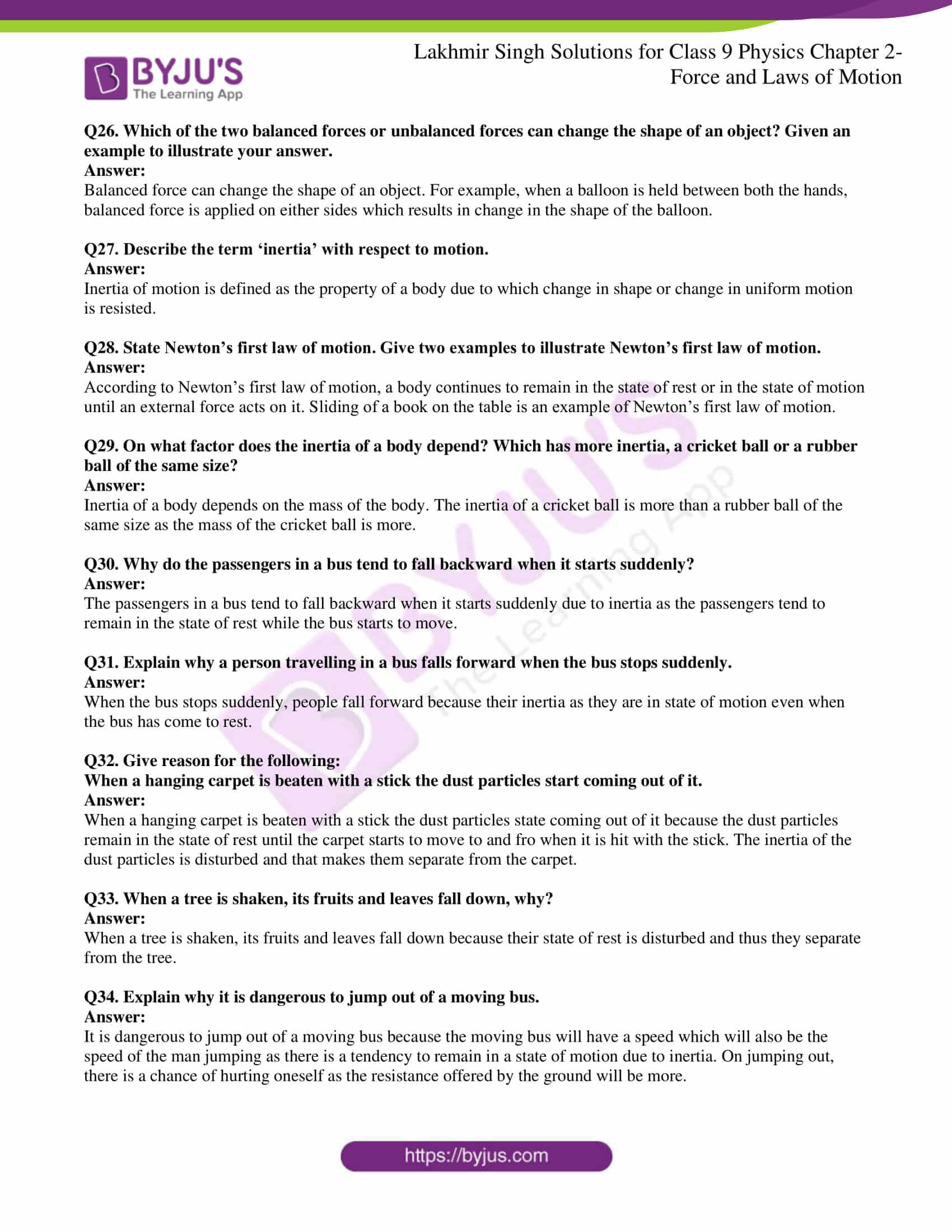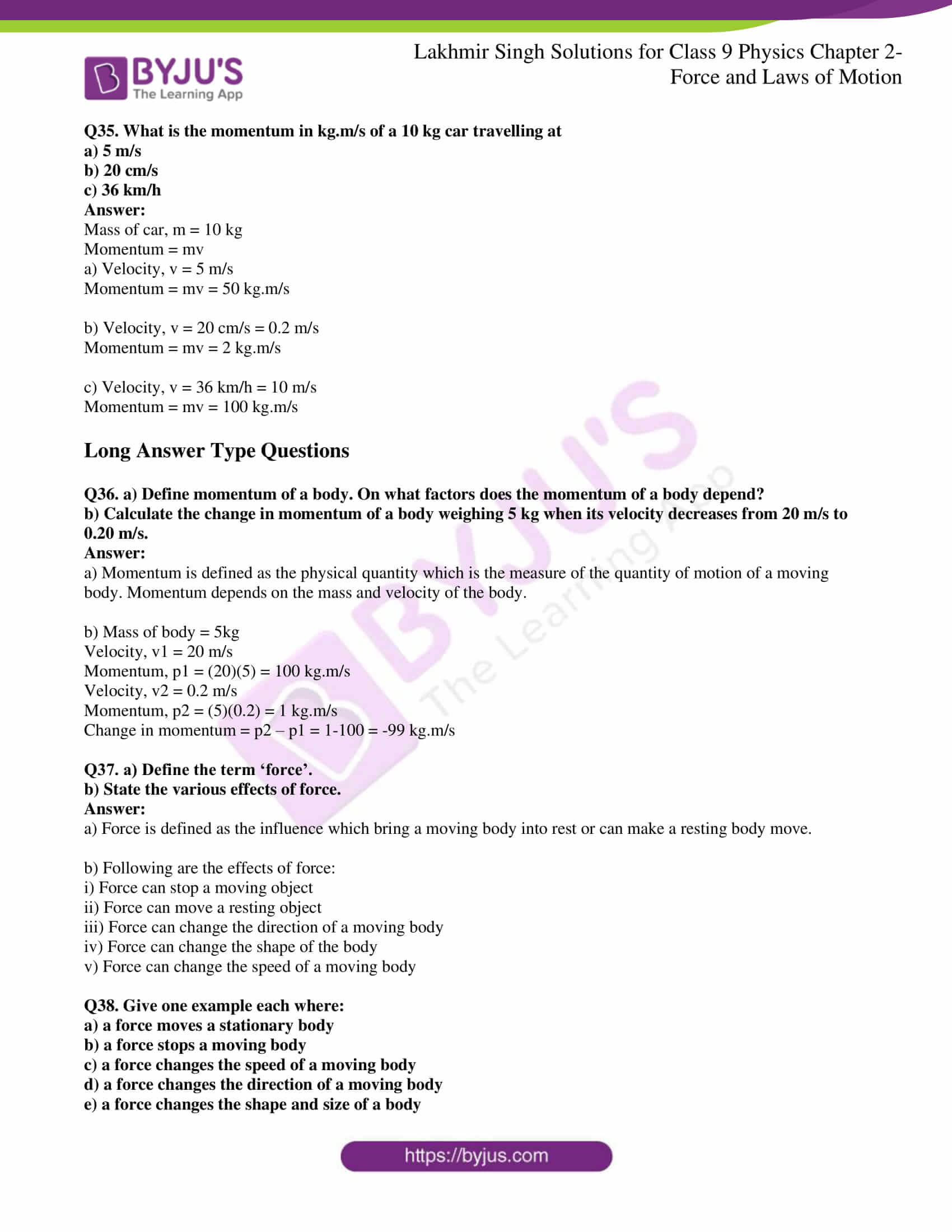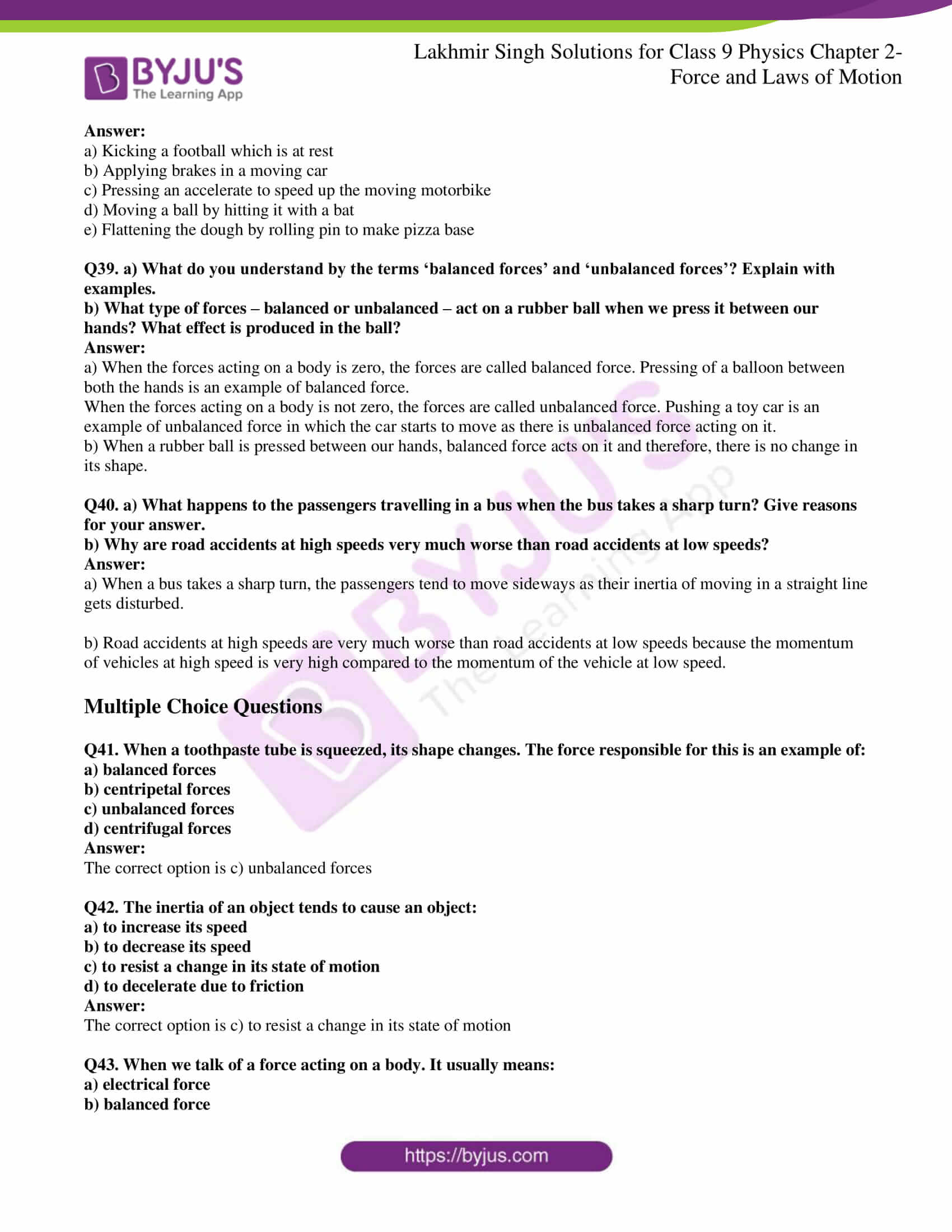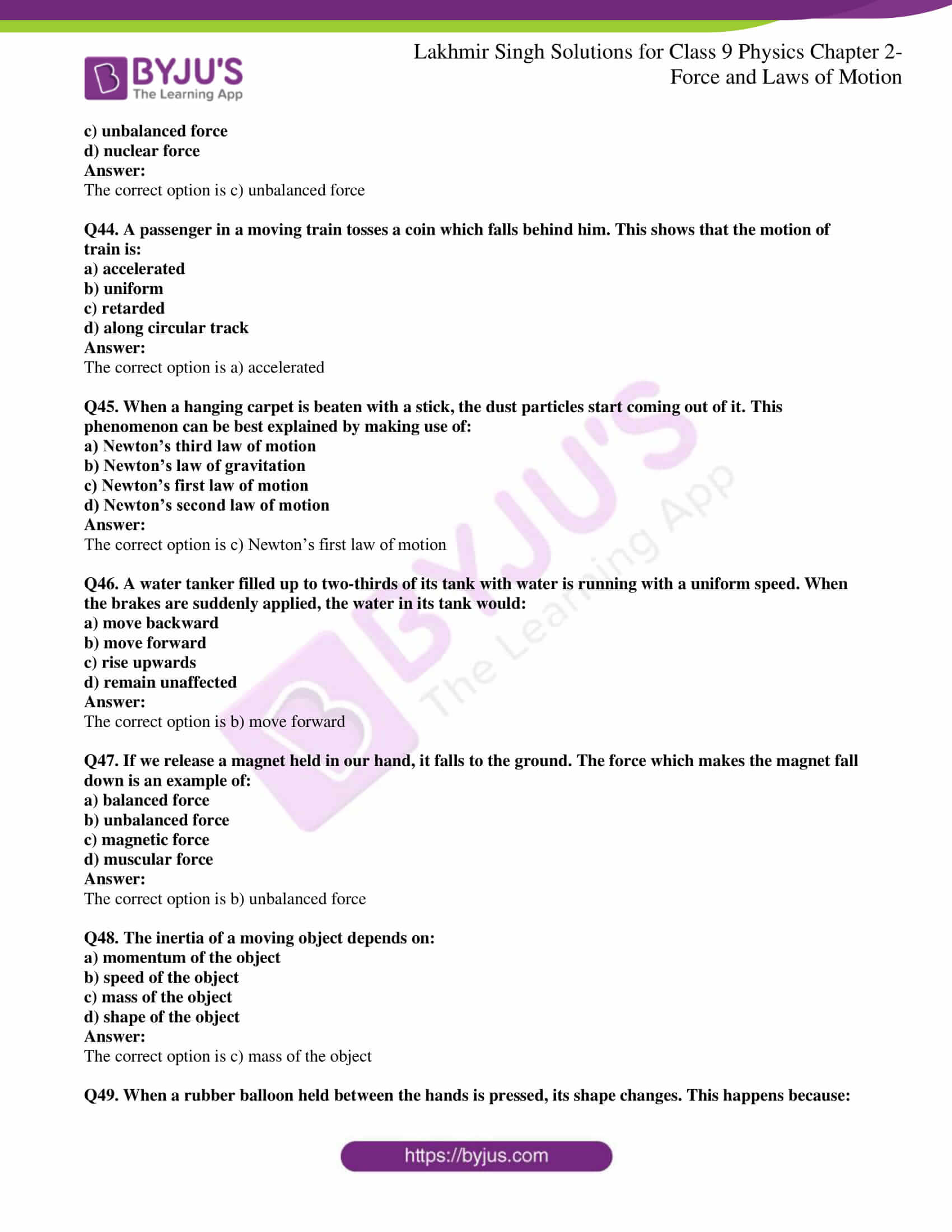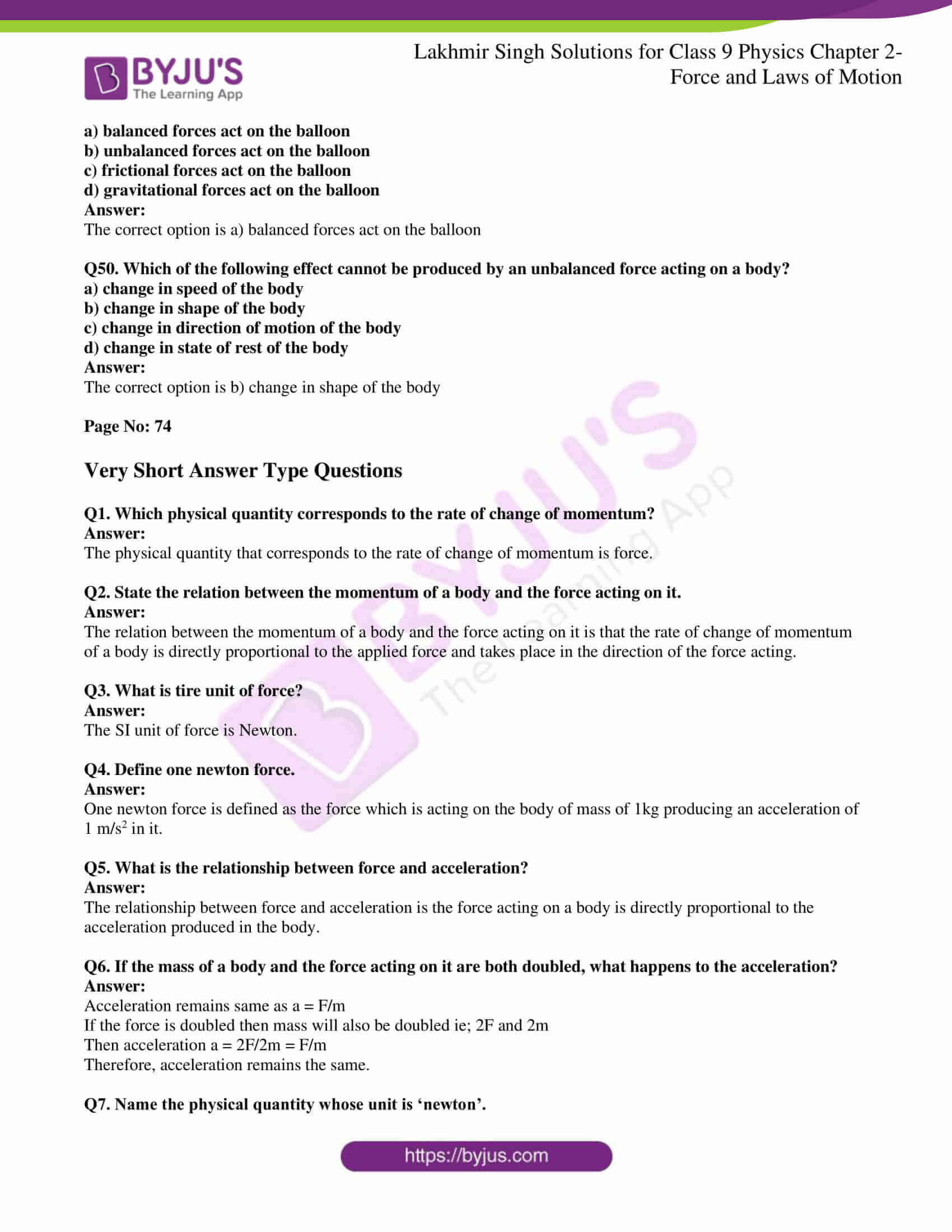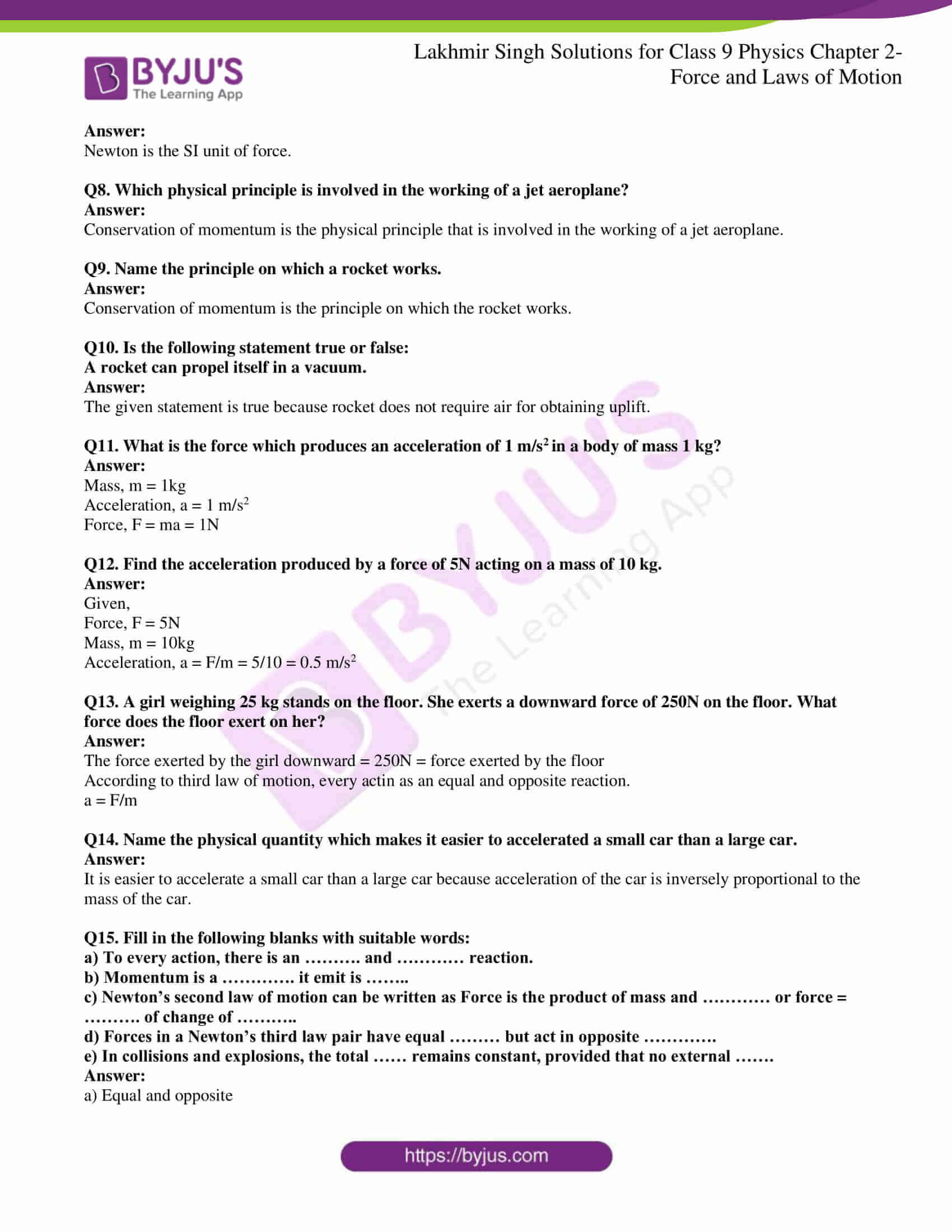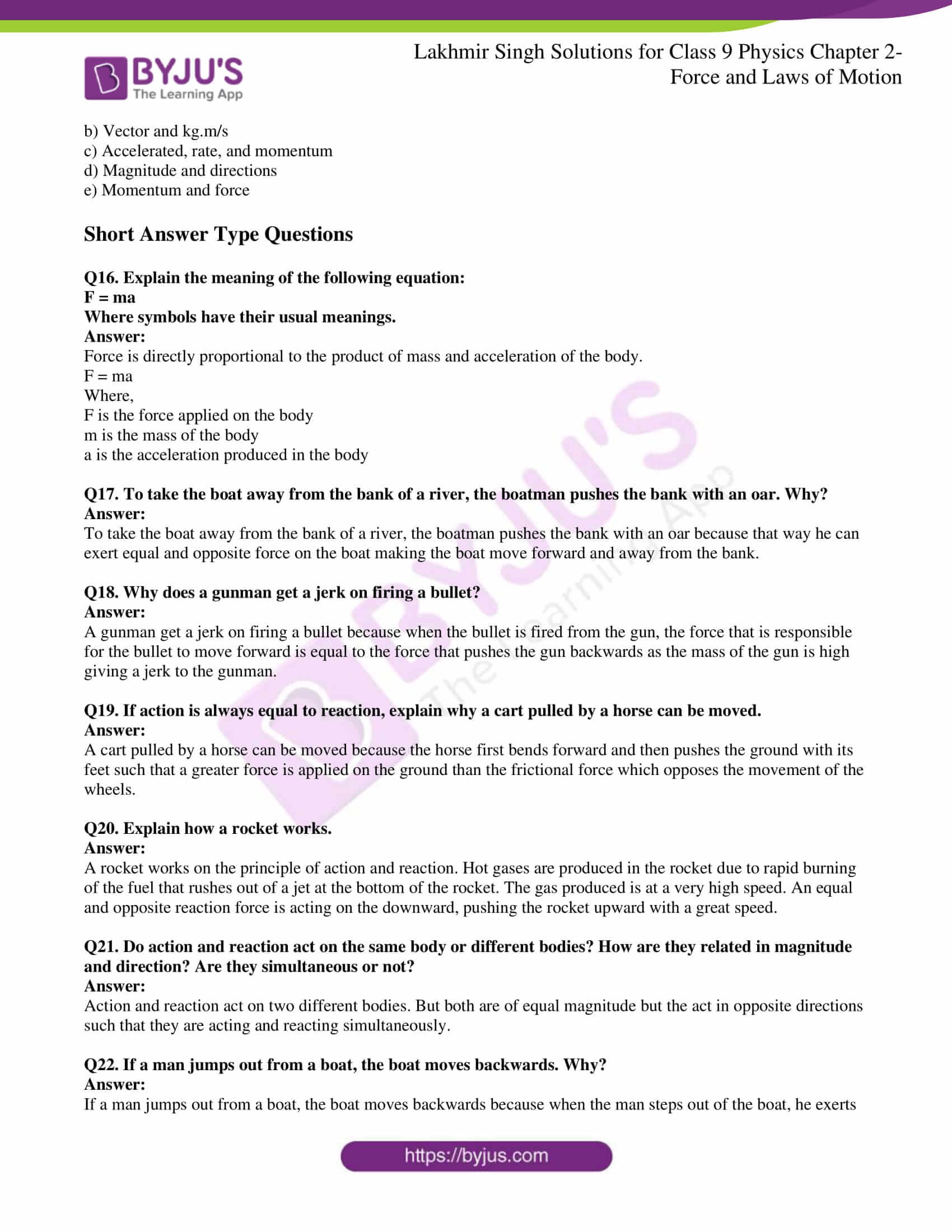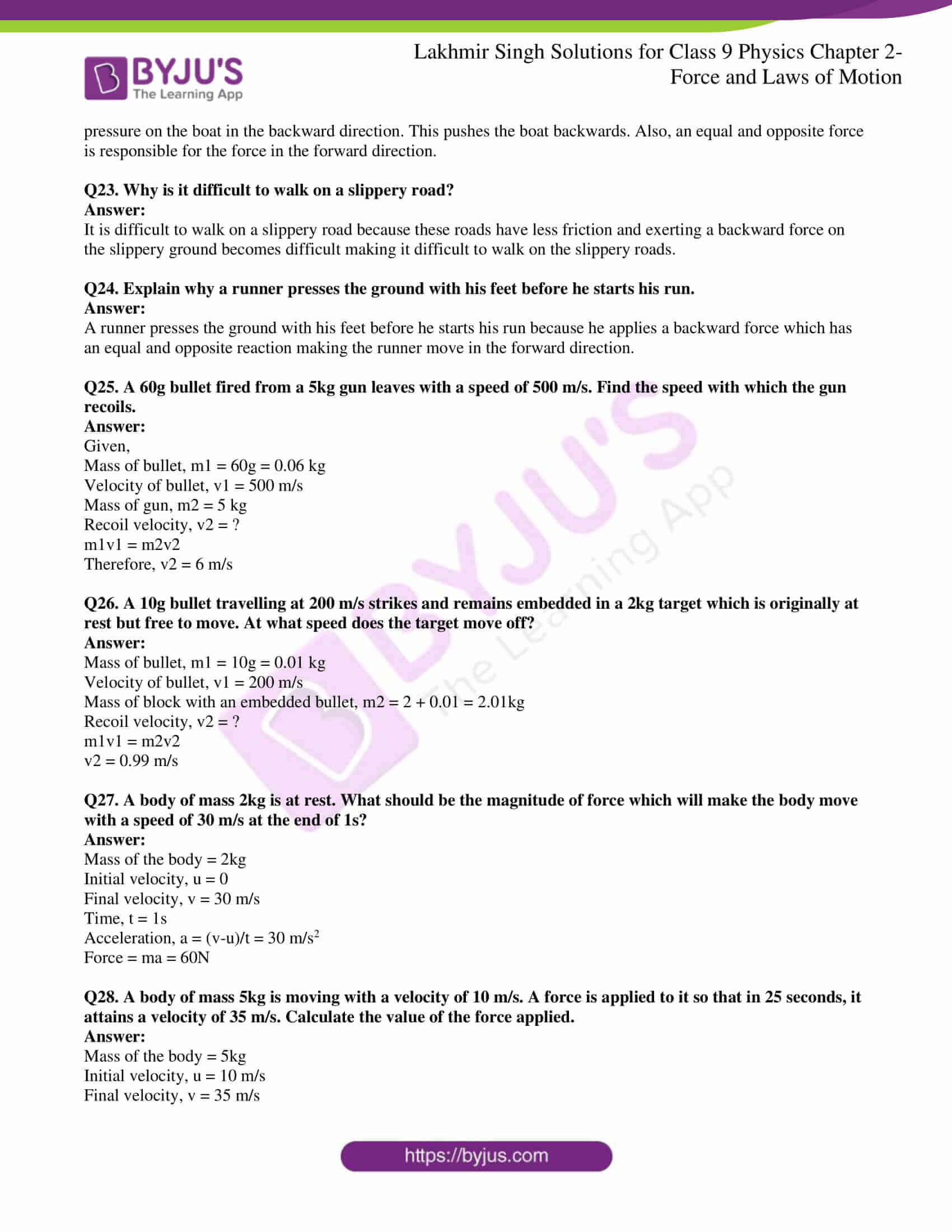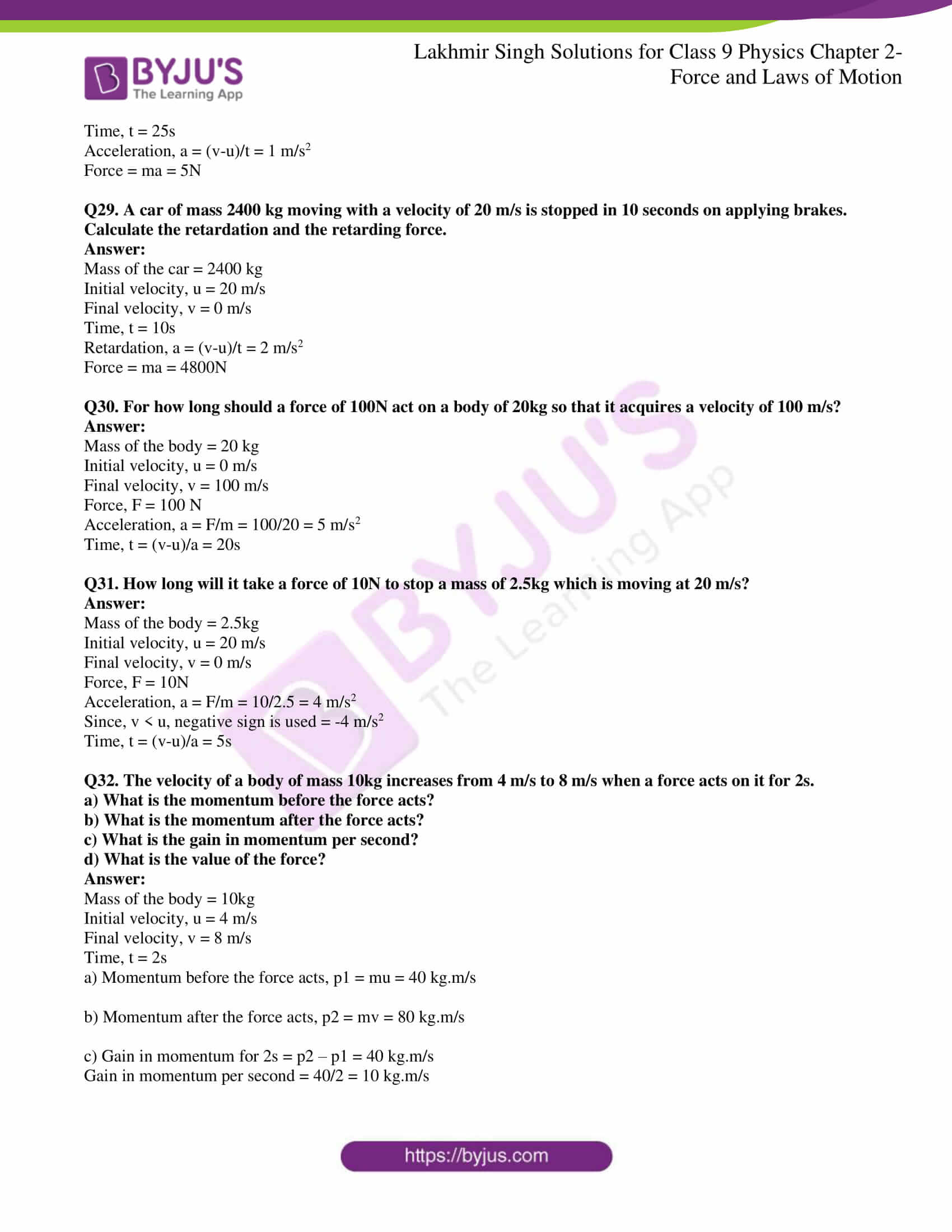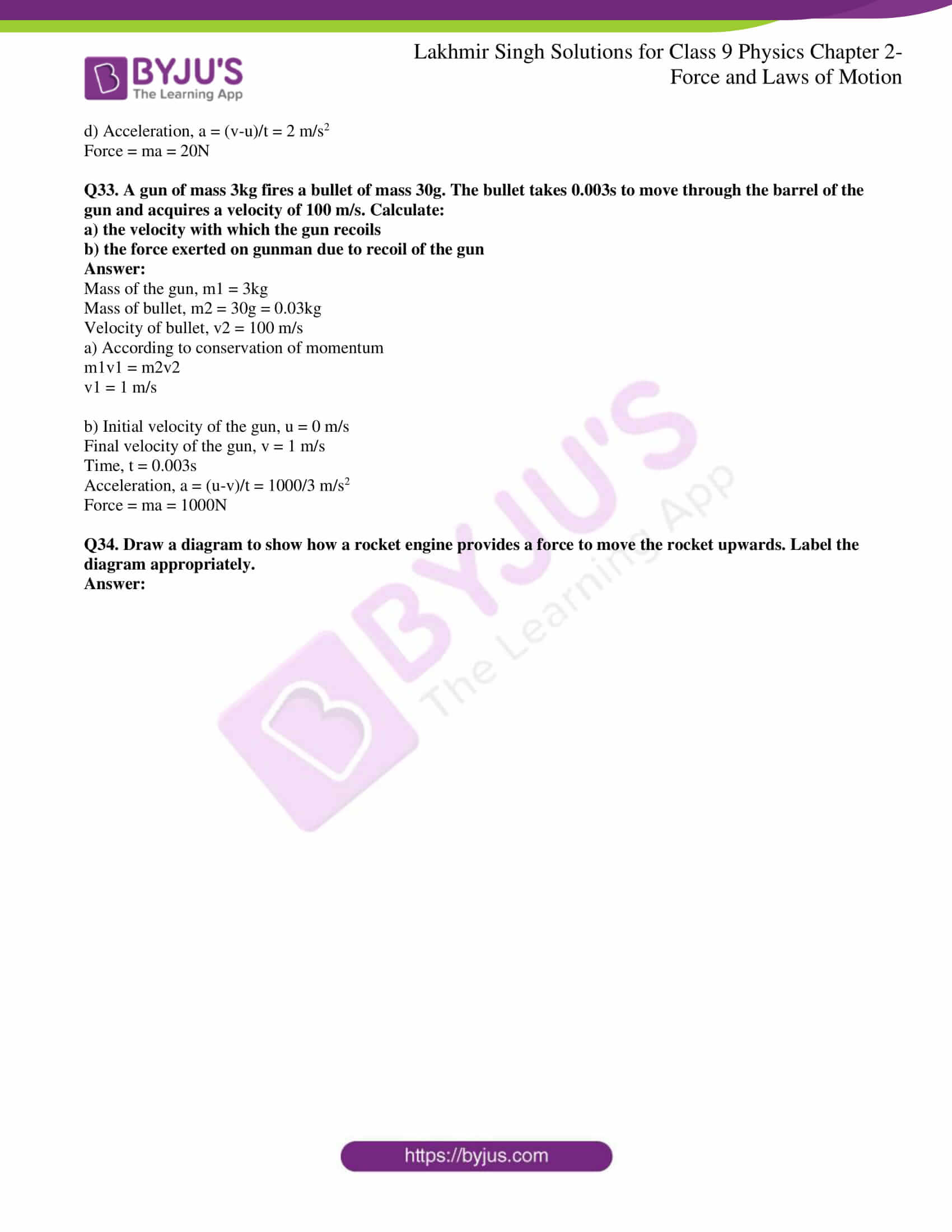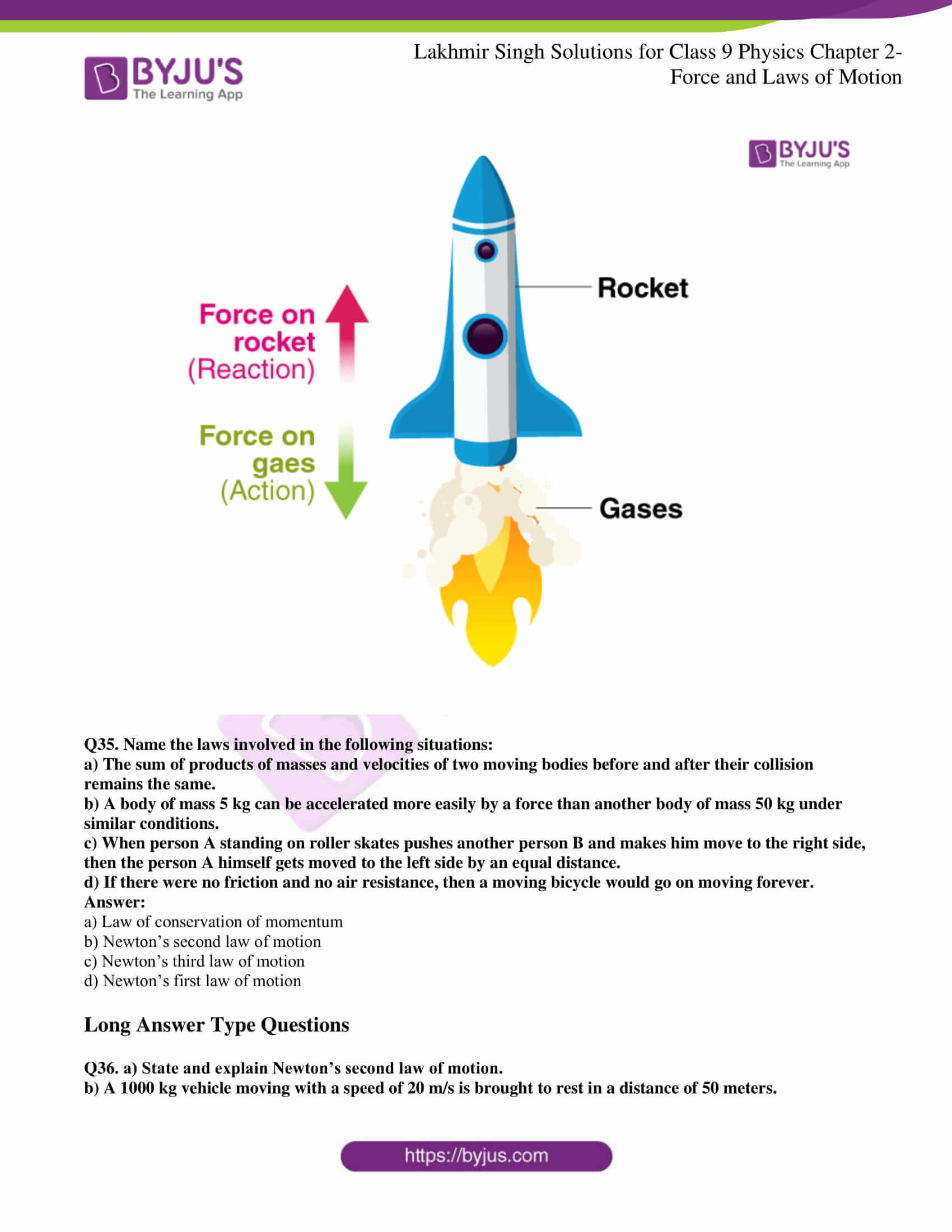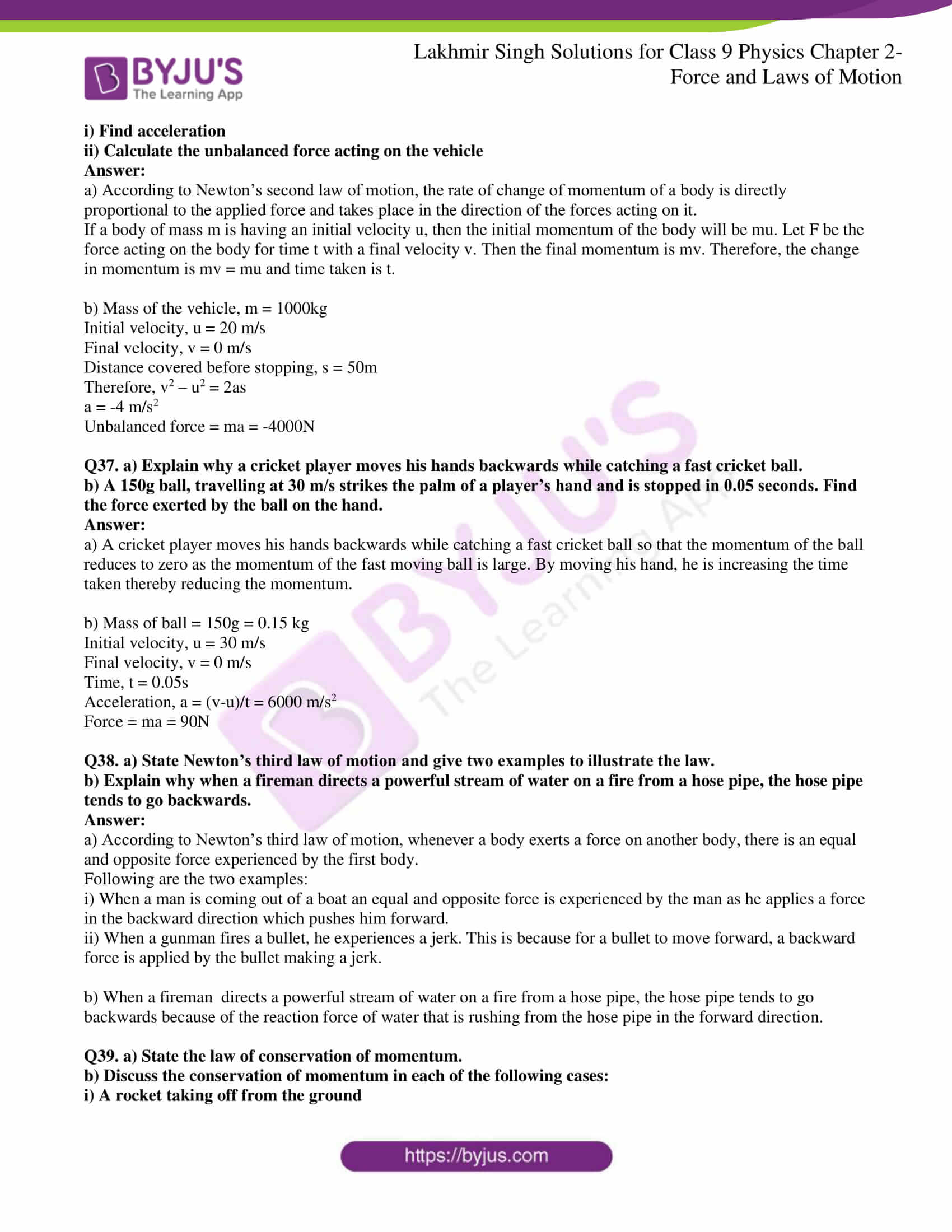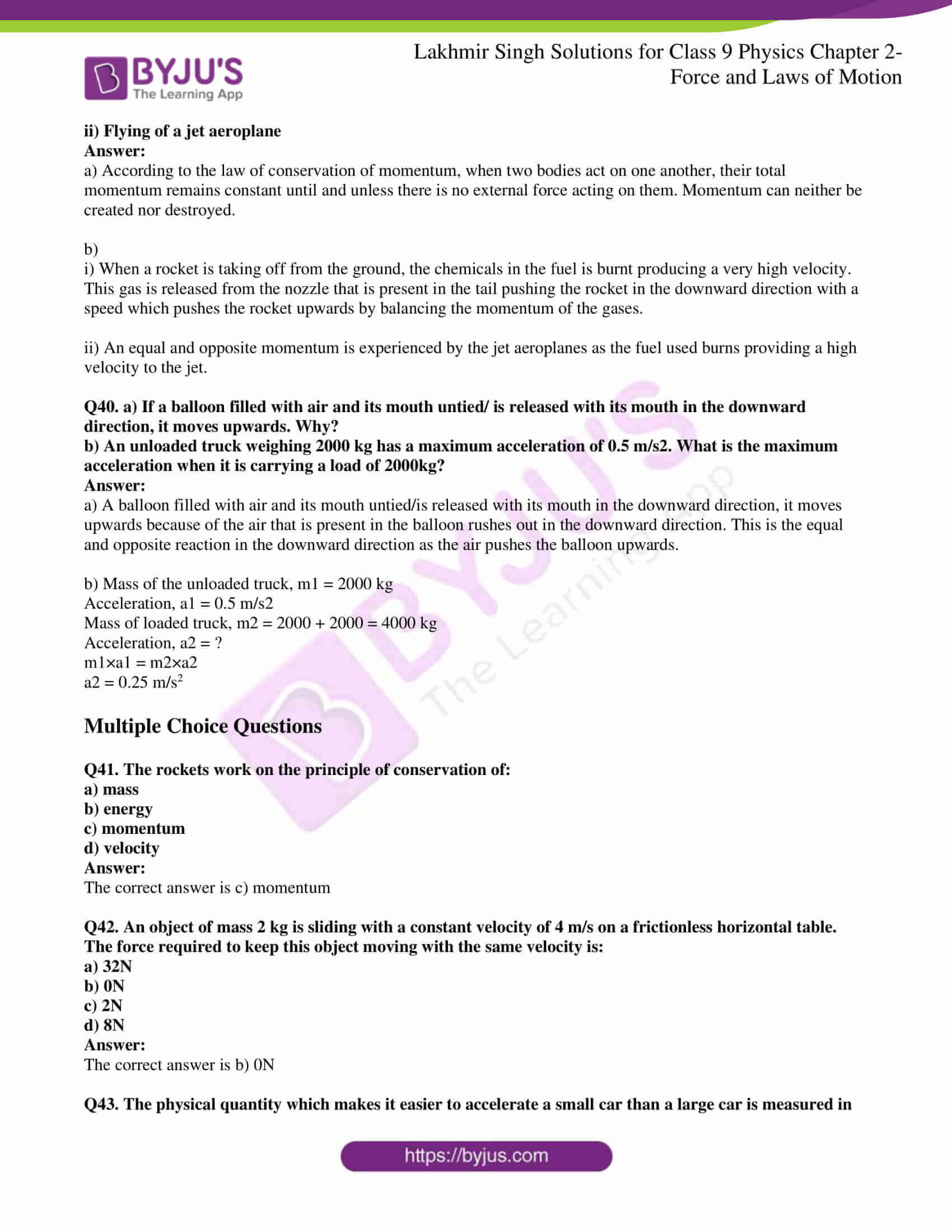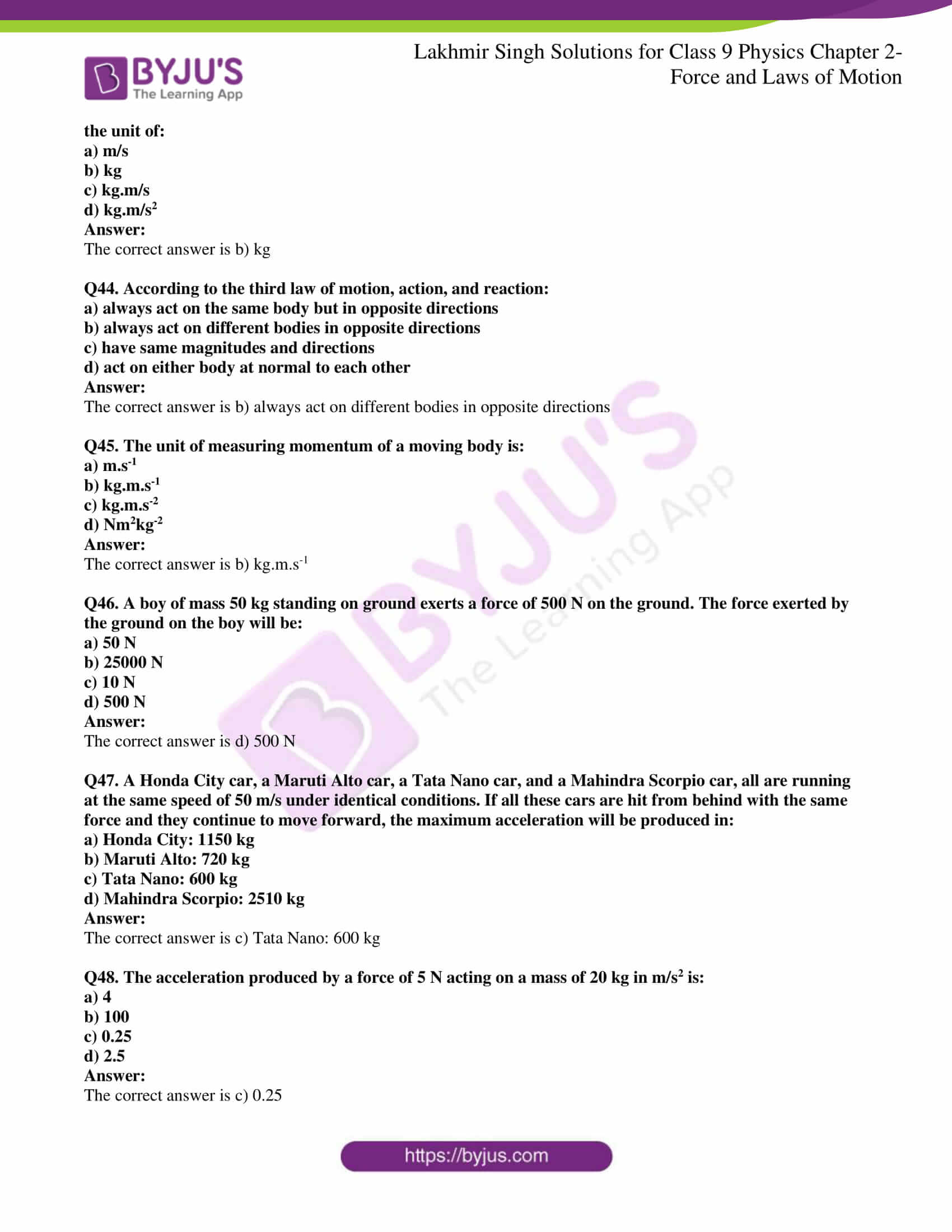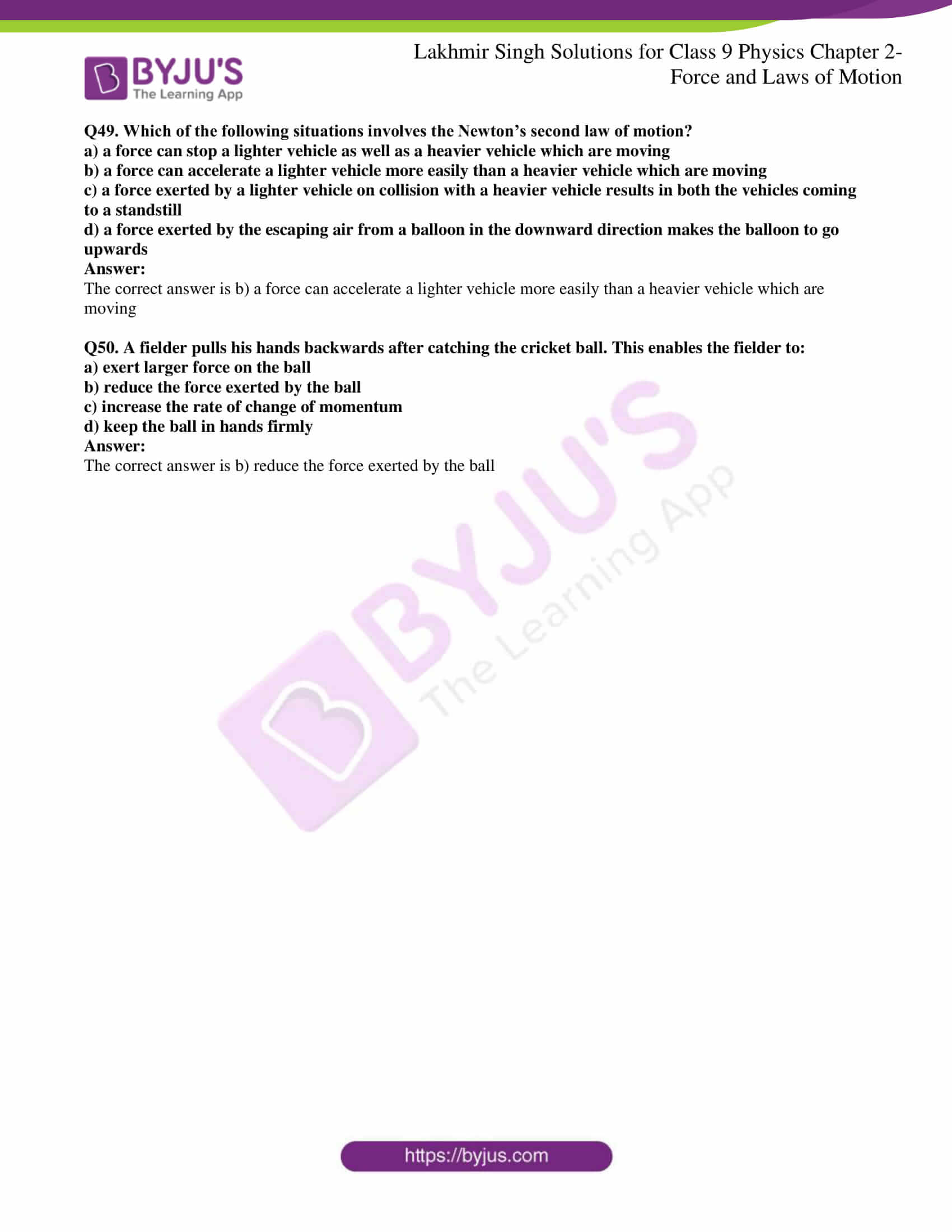Page No: 55

### Very Short Answer Type Questions

Q1. What name is given to the product of mass and velocity of a body?

The product of mass and velocity of a body is known as momentum.

Q2. Name the physical quantity which is considered to be a measure of the quantity of motion of a body.

Momentum is used for measuring the motion of a body.

Q3. What is the SI unit of momentum?

The SI unit of momentum is kg.m/s.

Q4. State whether momentum is scalar or vector.

Momentum is a vector quantity as it has magnitude as well as direction.

Q5. What is the total momentum of the bullet and the gun before firing?

The total momentum of the bullet and the gun before firing is zero as the velocities of them is zero.

Q6. Name the physical quantity whose unit is kg.m/s.

Momentum is the quantity whose unit is kg.m/s.

Q7. What will be the momentum of a body of mass ‘m’ which is moving with the velocity V?

Momentum of a body of mass m which is moving with the velocity V is given as:

p = mv

Q8. What is the usual name of the forces which cannot produce motion in a body but only change its shape?

Balanced force cannot produce motion in a body but can only change the shape.

Q9. Name the unbalanced force which slows down a moving bicycle when we stop pedaling it.

The unbalanced force which slows down a moving bicycle when we stop pedaling is known as frictional force.

Q10. State whether the following statement is true or false:

Unbalanced forces acting on a body change its shape.

False.

Q11. When a ball is dropped from a height, its speed increases gradually. Name the force which causes this change in speed.

The change in the speed of the ball is due to force of gravity.

Q12. Name the property of bodies to resist a change in their state of rest or of motion.

Inertia is responsible for the bodies to resist a change in their state of rest or of motion.

Q13. What is the other name of Newton’s first law of motion?

The other name is Galileo’s law of inertia.

Q14. The mass of object A is 6kg whereas that of another object B is 34 kg. Which of the two objects A or B has more inertia?

Object B has more inertia as the mass of object B is more than A.

Q15. Name the scientist who gave the laws of motion.

Sir Isaac Newton gave the laws of motion.

Q16. State whether force is a scalar or a vector quantity.

Force is a vector quantity.

Q17. With which physical quantity should the speed of a running bull be multiplied so as to obtain its momentum?

The speed of the running bull should be multiplied with its mass to get momentum

Q18. Fill in the blanks:

a) ……… is a measure of the inertia of a body.

b) When a running car stops suddenly, the passengers are jerked ……….

c) When a stationary car starts suddenly, the passengers are jerked ………

d) Newton’s first law of motion is also called Galileo’s law of ……….

e) If there were no unbalanced force of …… and no ………. resistance, a moving bicycle would go on moving forever.

a) Mass

b) Forward

c) Backward

d) Inertia

e) Friction and air

### Short Answer Type Questions

Q19. Explain why it is easier to stop a tennis ball than a cricket ball moving with the same speed.

It is easier to stop a tennis ball than a cricket ball moving with the same speed because the momentum of cricket ball will be higher than the tennis ball as the mass of the cricket ball is more than tennis ball. Therefore, less force is required to stop the tennis ball when compared to the cricket ball.

Q20. Explain the meaning of the following equation:

p = mv

where symbols have their usual meanings.

p = mv is the equation of momentum of a body which is equal to the product of its mass and velocity.

Where,

p is the momentum of the body

m is the mass of the body

v is the velocity of the body

Q21. Explain how a karate player can break a pile of tiles with a single blow of his hand.

A karate player can break a pile of tiles with a single blow of his hand because the force exerted by him is large enough to break these tiles as the momentum of his land is reduced to zero.

Q22. Calculate the momentum of a toy car of mass 200g moving with a speed of 5m/s.

Mass of the toy car, m = 200g = 0.2kg

Speed, v = 5 m/s

Momentum, p = mv = 1kg.m/s

Q23. What is the change in momentum of a car weighing 1500 kg when its speed increases from 36 km/h to 72 km/h uniformly?

Mass of car = 1500 kg

Velocity, v1 = 36 km/h = 10 m/s

Momentum, p1 = (1500)(10) = 15000 kg.m/s

Velocity, v2 = 72 km/h = 20 m/s

Momentum, p2 = (1500)(20) = 30000kg.m/s

Change in momentum = p2 – p1 = 15000 kg.m/s

Q24. A body of mass 25 kg has a momentum of 125 kg.m/s. Calculate the velocity of the body.

Mass of the body, m = 25kg

Momentum, p = 125 kg.m/s

p = mv

v = p/m = 5 m/s

Velocity of the body is 5 m/s

Q25. Calculate the momentum of the following:

a) an elephant of mass 2000kg moving at 5 m/s

b) a bullet of mass 0.02 kg moving at 400 m/s

a) Mass of elephant = 2000kg

Velocity = 5 m/s

Momentum = mv = 10000 kg.m/s

b) Mass of bullet = 0.02kg

Velocity = 400 m/s

Momentum = mv = 8 kg.m/s

Q26. Which of the two balanced forces or unbalanced forces can change the shape of an object? Given an example to illustrate your answer.

Balanced force can change the shape of an object. For example, when a balloon is held between both the hands, balanced force is applied on either sides which results in change in the shape of the balloon.

Q27. Describe the term ‘inertia’ with respect to motion.

Inertia of motion is defined as the property of a body due to which change in shape or change in uniform motion is resisted.

Q28. State Newton’s first law of motion. Give two examples to illustrate Newton’s first law of motion.

According to Newton’s first law of motion, a body continues to remain in the state of rest or in the state of motion until an external force acts on it. Sliding of a book on the table is an example of Newton’s first law of motion.

Q29. On what factor does the inertia of a body depend? Which has more inertia, a cricket ball or a rubber ball of the same size?

Inertia of a body depends on the mass of the body. The inertia of a cricket ball is more than a rubber ball of the same size as the mass of the cricket ball is more.

Q30. Why do the passengers in a bus tend to fall backward when it starts suddenly?

The passengers in a bus tend to fall backward when it starts suddenly due to inertia as the passengers tend to remain in the state of rest while the bus starts to move.

Q31. Explain why a person travelling in a bus falls forward when the bus stops suddenly.

When the bus stops suddenly, people fall forward because their inertia as they are in state of motion even when the bus has come to rest.

Q32. Give reason for the following:

When a hanging carpet is beaten with a stick the dust particles start coming out of it.

When a hanging carpet is beaten with a stick the dust particles state coming out of it because the dust particles remain in the state of rest until the carpet starts to move to and fro when it is hit with the stick. The inertia of the dust particles is disturbed and that makes them separate from the carpet.

Q33. When a tree is shaken, its fruits and leaves fall down, why?

When a tree is shaken, its fruits and leaves fall down because their state of rest is disturbed and thus they separate from the tree.

Q34. Explain why it is dangerous to jump out of a moving bus.

It is dangerous to jump out of a moving bus because the moving bus will have a speed which will also be the speed of the man jumping as there is a tendency to remain in a state of motion due to inertia. On jumping out, there is a chance of hurting oneself as the resistance offered by the ground will be more.

Q35. What is the momentum in kg.m/s of a 10 kg car travelling at

a) 5 m/s

b) 20 cm/s

c) 36 km/h

Mass of car, m = 10 kg

Momentum = mv

a) Velocity, v = 5 m/s

Momentum = mv = 50 kg.m/s

b) Velocity, v = 20 cm/s = 0.2 m/s

Momentum = mv = 2 kg.m/s

c) Velocity, v = 36 km/h = 10 m/s

Momentum = mv = 100 kg.m/s

### Long Answer Type Questions

Q36. a) Define momentum of a body. On what factors does the momentum of a body depend?

b) Calculate the change in momentum of a body weighing 5 kg when its velocity decreases from 20 m/s to 0.20 m/s.

a) Momentum is defined as the physical quantity which is the measure of the quantity of motion of a moving body. Momentum depends on the mass and velocity of the body.

b) Mass of body = 5kg

Velocity, v1 = 20 m/s

Momentum, p1 = (20)(5) = 100 kg.m/s

Velocity, v2 = 0.2 m/s

Momentum, p2 = (5)(0.2) = 1 kg.m/s

Change in momentum = p2 – p1 = 1-100 = -99 kg.m/s

Q37. a) Define the term ‘force’.

b) State the various effects of force.

a) Force is defined as the influence which bring a moving body into rest or can make a resting body move.

b) Following are the effects of force:

i) Force can stop a moving object

ii) Force can move a resting object

iii) Force can change the direction of a moving body

iv) Force can change the shape of the body

v) Force can change the speed of a moving body

Q38. Give one example each where:

a) a force moves a stationary body

b) a force stops a moving body

c) a force changes the speed of a moving body

d) a force changes the direction of a moving body

e) a force changes the shape and size of a body

a) Kicking a football which is at rest

b) Applying brakes in a moving car

c) Pressing an accelerate to speed up the moving motorbike

d) Moving a ball by hitting it with a bat

e) Flattening the dough by rolling pin to make pizza base

Q39. a) What do you understand by the terms ‘balanced forces’ and ‘unbalanced forces’? Explain with examples.

b) What type of forces – balanced or unbalanced – act on a rubber ball when we press it between our hands? What effect is produced in the ball?

a) When the forces acting on a body is zero, the forces are called balanced force. Pressing of a balloon between both the hands is an example of balanced force.

When the forces acting on a body is not zero, the forces are called unbalanced force. Pushing a toy car is an example of unbalanced force in which the car starts to move as there is unbalanced force acting on it.

b) When a rubber ball is pressed between our hands, balanced force acts on it and therefore, there is no change in its shape.

Q40. a) What happens to the passengers travelling in a bus when the bus takes a sharp turn? Give reasons for your answer.

b) Why are road accidents at high speeds very much worse than road accidents at low speeds?

a) When a bus takes a sharp turn, the passengers tend to move sideways as their inertia of moving in a straight line gets disturbed.

b) Road accidents at high speeds are very much worse than road accidents at low speeds because the momentum of vehicles at high speed is very high compared to the momentum of the vehicle at low speed.

### Multiple Choice Questions

Q41. When a toothpaste tube is squeezed, its shape changes. The force responsible for this is an example of:

a) balanced forces

b) centripetal forces

c) unbalanced forces

d) centrifugal forces

The correct option is c) unbalanced forces

Q42. The inertia of an object tends to cause an object:

a) to increase its speed

b) to decrease its speed

c) to resist a change in its state of motion

d) to decelerate due to friction

The correct option is c) to resist a change in its state of motion

Q43. When we talk of a force acting on a body. It usually means:

a) electrical force

b) balanced force

c) unbalanced force

d) nuclear force

The correct option is c) unbalanced force

Q44. A passenger in a moving train tosses a coin which falls behind him. This shows that the motion of train is:

a) accelerated

b) uniform

c) retarded

d) along circular track

The correct option is a) accelerated

Q45. When a hanging carpet is beaten with a stick, the dust particles start coming out of it. This phenomenon can be best explained by making use of:

a) Newton’s third law of motion

b) Newton’s law of gravitation

c) Newton’s first law of motion

d) Newton’s second law of motion

The correct option is c) Newton’s first law of motion

Q46. A water tanker filled up to two-thirds of its tank with water is running with a uniform speed. When the brakes are suddenly applied, the water in its tank would:

a) move backward

b) move forward

c) rise upwards

d) remain unaffected

The correct option is b) move forward

Q47. If we release a magnet held in our hand, it falls to the ground. The force which makes the magnet fall down is an example of:

a) balanced force

b) unbalanced force

c) magnetic force

d) muscular force

The correct option is b) unbalanced force

Q48. The inertia of a moving object depends on:

a) momentum of the object

b) speed of the object

c) mass of the object

d) shape of the object

The correct option is c) mass of the object

Q49. When a rubber balloon held between the hands is pressed, its shape changes. This happens because:

a) balanced forces act on the balloon

b) unbalanced forces act on the balloon

c) frictional forces act on the balloon

d) gravitational forces act on the balloon

The correct option is a) balanced forces act on the balloon

Q50. Which of the following effect cannot be produced by an unbalanced force acting on a body?

a) change in speed of the body

b) change in shape of the body

c) change in direction of motion of the body

d) change in state of rest of the body

The correct option is b) change in shape of the body

Page No: 74

### Very Short Answer Type Questions

Q1. Which physical quantity corresponds to the rate of change of momentum?

The physical quantity that corresponds to the rate of change of momentum is force.

Q2. State the relation between the momentum of a body and the force acting on it.

The relation between the momentum of a body and the force acting on it is that the rate of change of momentum of a body is directly proportional to the applied force and takes place in the direction of the force acting.

Q3. What is the unit of force?

The SI unit of force is Newton.

Q4. Define one newton force.

One newton force is defined as the force which is acting on the body of mass of 1kg producing an acceleration of 1 m/s2 in it.

Q5. What is the relationship between force and acceleration?

The relationship between force and acceleration is the force acting on a body is directly proportional to the acceleration produced in the body.

Q6. If the mass of a body and the force acting on it are both doubled, what happens to the acceleration?

Acceleration remains same as a = F/m

If the force is doubled then mass will also be doubled ie; 2F and 2m

Then acceleration a = 2F/2m = F/m

Therefore, acceleration remains the same.

Q7. Name the physical quantity whose unit is ‘newton’.

Newton is the SI unit of force.

Q8. Which physical principle is involved in the working of a jet aeroplane?

Conservation of momentum is the physical principle that is involved in the working of a jet aeroplane.

Q9. Name the principle on which a rocket works.

Conservation of momentum is the principle on which the rocket works.

Q10. Is the following statement true or false:

A rocket can propel itself in a vacuum.

The given statement is true because rocket does not require air for obtaining uplift.

Q11. What is the force which produces an acceleration of 1 m/s2 in a body of mass 1 kg?

Mass, m = 1kg

Acceleration, a = 1 m/s2

Force, F = ma = 1N

Q12. Find the acceleration produced by a force of 5N acting on a mass of 10 kg.

Given,

Force, F = 5N

Mass, m = 10kg

Acceleration, a = F/m = 5/10 = 0.5 m/s2

Q13. A girl weighing 25 kg stands on the floor. She exerts a downward force of 250N on the floor. What force does the floor exert on her?

The force exerted by the girl downward = 250N = force exerted by the floor

According to third law of motion, every actin as an equal and opposite reaction.

a = F/m

Q14. Name the physical quantity which makes it easier to accelerated a small car than a large car.

It is easier to accelerate a small car than a large car because acceleration of the car is inversely proportional to the mass of the car.

Q15. Fill in the following blanks with suitable words:

a) To every action, there is an ………. and ………… reaction.

b) Momentum is a …………. it emit is ……..

c) Newton’s second law of motion can be written as Force is the product of mass and ………… or force = ………. of change of ………..

d) Forces in a Newton’s third law pair have equal ……… but act in opposite ………….

e) In collisions and explosions, the total …… remains constant, provided that no external …….

a) Equal and opposite

b) Vector and kg.m/s

c) Accelerated, rate, and momentum

d) Magnitude and directions

e) Momentum and force

### Short Answer Type Questions

Q16. Explain the meaning of the following equation:

F = ma

Where symbols have their usual meanings.

Force is directly proportional to the product of mass and acceleration of the body.

F = ma

Where,

F is the force applied on the body

m is the mass of the body

a is the acceleration produced in the body

Q17. To take the boat away from the bank of a river, the boatman pushes the bank with an oar. Why?

To take the boat away from the bank of a river, the boatman pushes the bank with an oar because that way he can exert equal and opposite force on the boat making the boat move forward and away from the bank.

Q18. Why does a gunman get a jerk on firing a bullet?

A gunman get a jerk on firing a bullet because when the bullet is fired from the gun, the force that is responsible for the bullet to move forward is equal to the force that pushes the gun backwards as the mass of the gun is high giving a jerk to the gunman.

Q19. If action is always equal to reaction, explain why a cart pulled by a horse can be moved.

A cart pulled by a horse can be moved because the horse first bends forward and then pushes the ground with its feet such that a greater force is applied on the ground than the frictional force, which opposes the movement of the wheels.

Q20. Explain how a rocket works.

A rocket works on the principle of action and reaction. Hot gases are produced in the rocket due to rapid burning of the fuel that rushes out of a jet at the bottom of the rocket. The gas produced is at a very high speed. An equal and opposite reaction force is acting on the downward, pushing the rocket upward with a great speed.

Q21. Do action and reaction act on the same body or different bodies? How are they related in magnitude and direction? Are they simultaneous or not?

Action and reaction act on two different bodies. But both are of equal magnitude but the act in opposite directions such that they are acting and reacting simultaneously.

Q22. If a man jumps out from a boat, the boat moves backwards. Why?

If a man jumps out from a boat, the boat moves backwards because when the man steps out of the boat, he exerts pressure on the boat in the backward direction. This pushes the boat backwards. Also, an equal and opposite force is responsible for the force in the forward direction.

Q23. Why is it difficult to walk on a slippery road?

It is difficult to walk on a slippery road because these roads have less friction and exerting a backward force on the slippery ground becomes difficult making it difficult to walk on the slippery roads.

Q24. Explain why a runner presses the ground with his feet before he starts his run.

A runner presses the ground with his feet before he starts his run because he applies a backward force which has an equal and opposite reaction making the runner move in the forward direction.

Q25. A 60g bullet fired from a 5kg gun leaves with a speed of 500 m/s. Find the speed with which the gun recoils.

Given,

Mass of bullet, m1 = 60g = 0.06 kg

Velocity of bullet, v1 = 500 m/s

Mass of gun, m2 = 5 kg

Recoil velocity, v2 = ?

m1v1 = m2v2

Therefore, v2 = 6 m/s

Q26. A 10g bullet travelling at 200 m/s strikes and remains embedded in a 2kg target which is originally at rest but free to move. At what speed does the target move off?

Mass of bullet, m1 = 10g = 0.01 kg

Velocity of bullet, v1 = 200 m/s

Mass of block with an embedded bullet, m2 = 2 + 0.01 = 2.01kg

Recoil velocity, v2 = ?

m1v1 = m2v2

v2 = 0.99 m/s

Q27. A body of mass 2kg is at rest. What should be the magnitude of force which will make the body move with a speed of 30 m/s at the end of 1s?

Mass of the body = 2kg

Initial velocity, u = 0

Final velocity, v = 30 m/s

Time, t = 1s

Acceleration, a = (v-u)/t = 30 m/s2

Force = ma = 60N

Q28. A body of mass 5kg is moving with a velocity of 10 m/s. A force is applied to it so that in 25 seconds, it attains a velocity of 35 m/s. Calculate the value of the force applied.

Mass of the body = 5kg

Initial velocity, u = 10 m/s

Final velocity, v = 35 m/s

Time, t = 25s

Acceleration, a = (v-u)/t = 1 m/s2

Force = ma = 5N

Q29. A car of mass 2400 kg moving with a velocity of 20 m/s is stopped in 10 seconds on applying brakes. Calculate the retardation and the retarding force.

Mass of the car = 2400 kg

Initial velocity, u = 20 m/s

Final velocity, v = 0 m/s

Time, t = 10s

Retardation, a = (v-u)/t = 2 m/s2

Force = ma = 4800N

Q30. For how long should a force of 100N act on a body of 20kg so that it acquires a velocity of 100 m/s?

Mass of the body = 20 kg

Initial velocity, u = 0 m/s

Final velocity, v = 100 m/s

Force, F = 100 N

Acceleration, a = F/m = 100/20 = 5 m/s2

Time, t = (v-u)/a = 20s

Q31. How long will it take a force of 10N to stop a mass of 2.5kg which is moving at 20 m/s?

Mass of the body = 2.5kg

Initial velocity, u = 20 m/s

Final velocity, v = 0 m/s

Force, F = 10N

Acceleration, a = F/m = 10/2.5 = 4 m/s2

Since, v < u, negative sign is used = -4 m/s2

Time, t = (v-u)/a = 5s

Q32. The velocity of a body of mass 10kg increases from 4 m/s to 8 m/s when a force acts on it for 2s.

a) What is the momentum before the force acts?

b) What is the momentum after the force acts?

c) What is the gain in momentum per second?

d) What is the value of the force?

Mass of the body = 10kg

Initial velocity, u = 4 m/s

Final velocity, v = 8 m/s

Time, t = 2s

a) Momentum before the force acts, p1 = mu = 40 kg.m/s

b) Momentum after the force acts, p2 = mv = 80 kg.m/s

c) Gain in momentum for 2s = p2 – p1 = 40 kg.m/s

Gain in momentum per second = 40/2 = 10 kg.m/s

d) Acceleration, a = (v-u)/t = 2 m/s2

Force = ma = 20N

Q33. A gun of mass 3kg fires a bullet of mass 30g. The bullet takes 0.003s to move through the barrel of the gun and acquires a velocity of 100 m/s. Calculate:

a) the velocity with which the gun recoils

b) the force exerted on gunman due to recoil of the gun

Mass of the gun, m1 = 3kg

Mass of bullet, m2 = 30g = 0.03kg

Velocity of bullet, v2 = 100 m/s

a) According to conservation of momentum

m1v1 = m2v2

v1 = 1 m/s

b) Initial velocity of the gun, u = 0 m/s

Final velocity of the gun, v = 1 m/s

Time, t = 0.003s

Acceleration, a = (u-v)/t = 1000/3 m/s2

Force = ma = 1000N

Q34. Draw a diagram to show how a rocket engine provides a force to move the rocket upwards. Label the diagram appropriately.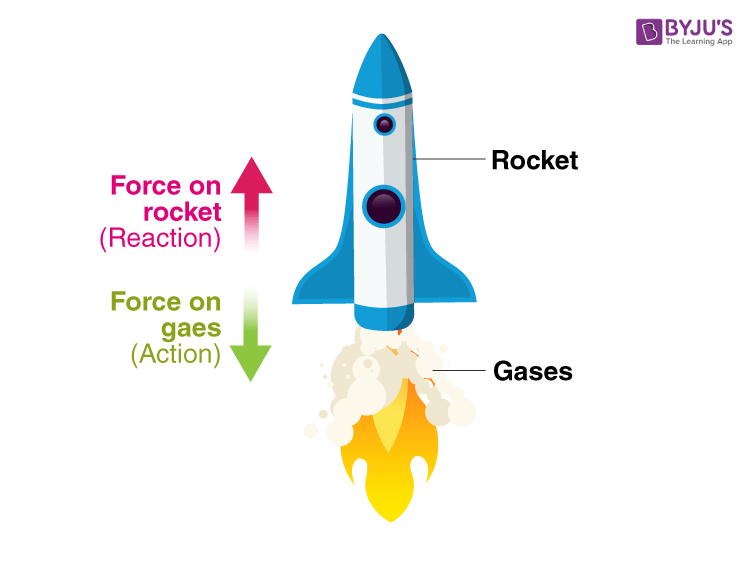Q35. Name the laws involved in the following situations:

a) The sum of products of masses and velocities of two moving bodies before and after their collision remains the same.

b) A body of mass 5 kg can be accelerated more easily by a force than another body of mass 50 kg under similar conditions.

c) When person A standing on roller skates pushes another person B and makes him move to the right side, then the person A himself gets moved to the left side by an equal distance.

d) If there were no friction and no air resistance, then a moving bicycle would go on moving forever.

a) Law of conservation of momentum

b) Newton’s second law of motion

c) Newton’s third law of motion

d) Newton’s first law of motion

### Long Answer Type Questions

Q36. a) State and explain Newton’s second law of motion.

b) A 1000 kg vehicle moving with a speed of 20 m/s is brought to rest in a distance of 50 meters.

i) Find acceleration

ii) Calculate the unbalanced force acting on the vehicle

a) According to Newton’s second law of motion, the rate of change of momentum of a body is directly proportional to the applied force and takes place in the direction of the forces acting on it.

If a body of mass m is having an initial velocity u, then the initial momentum of the body will be mu. Let F be the force acting on the body for time t with a final velocity v. Then the final momentum is mv. Therefore, the change in momentum is mv = mu and time taken is t.

b) Mass of the vehicle, m = 1000kg

Initial velocity, u = 20 m/s

Final velocity, v = 0 m/s

Distance covered before stopping, s = 50m

Therefore, v2 – u2 = 2as

a = -4 m/s2

Unbalanced force = ma = -4000N

Q37. a) Explain why a cricket player moves his hands backwards while catching a fast cricket ball.

b) A 150g ball, travelling at 30 m/s strikes the palm of a player’s hand and is stopped in 0.05 seconds. Find the force exerted by the ball on the hand.

a) A cricket player moves his hands backwards while catching a fast cricket ball so that the momentum of the ball reduces to zero as the momentum of the fast moving ball is large. By moving his hand, he is increasing the time taken thereby reducing the momentum.

b) Mass of ball = 150g = 0.15 kg

Initial velocity, u = 30 m/s

Final velocity, v = 0 m/s

Time, t = 0.05s

Acceleration, a = (v-u)/t = 6000 m/s2

Force = ma = 90N

Q38. a) State Newton’s third law of motion and give two examples to illustrate the law.

b) Explain why when a fireman directs a powerful stream of water on a fire from a hose pipe, the hose pipe tends to go backwards.

a) According to Newton’s third law of motion, whenever a body exerts a force on another body, there is an equal and opposite force experienced by the first body.

Following are the two examples:

i) When a man is coming out of a boat an equal and opposite force is experienced by the man as he applies a force in the backward direction which pushes him forward.

ii) When a gunman fires a bullet, he experiences a jerk. This is because for a bullet to move forward, a backward force is applied by the bullet making a jerk.

b) When a fireman directs a powerful stream of water on a fire from a hose pipe, the hose pipe tends to go backwards because of the reaction force of water that is rushing from the hose pipe in the forward direction.

Q39. a) State the law of conservation of momentum.

b) Discuss the conservation of momentum in each of the following cases:

i) A rocket taking off from the ground

ii) Flying of a jet aeroplane

a) According to the law of conservation of momentum, when two bodies act on one another, their total momentum remains constant until and unless there is no external force acting on them. Momentum can neither be created nor destroyed.

b)

i) When a rocket is taking off from the ground, the chemicals in the fuel is burnt producing a very high velocity. This gas is released from the nozzle that is present in the tail pushing the rocket in the downward direction with a speed which pushes the rocket upwards by balancing the momentum of the gases.

ii) An equal and opposite momentum is experienced by the jet aeroplanes as the fuel used burns providing a high velocity to the jet.

Q40. a) If a balloon filled with air and its mouth untied/ is released with its mouth in the downward direction, it moves upwards. Why?

b) An unloaded truck weighing 2000 kg has a maximum acceleration of 0.5 m/s2. What is the maximum acceleration when it is carrying a load of 2000kg?

a) A balloon filled with air and its mouth untied/is released with its mouth in the downward direction, it moves upwards because of the air that is present in the balloon rushes out in the downward direction. This is the equal and opposite reaction in the downward direction as the air pushes the balloon upwards.

b) Mass of the unloaded truck, m1 = 2000 kg

Acceleration, a1 = 0.5 m/s2

Mass of loaded truck, m2 = 2000 + 2000 = 4000 kg

Acceleration, a2 = ?

m1×a1 = m2×a2

a2 = 0.25 m/s2

### Multiple Choice Questions

Q41. The rockets work on the principle of conservation of:

a) mass

b) energy

c) momentum

d) velocity

The correct answer is c) momentum

Q42. An object of mass 2 kg is sliding with a constant velocity of 4 m/s on a frictionless horizontal table. The force required to keep this object moving with the same velocity is:

a) 32N

b) 0N

c) 2N

d) 8N

The correct answer is b) 0N

Q43. The physical quantity which makes it easier to accelerate a small car than a large car is measured in the unit of:

a) m/s

b) kg

c) kg.m/s

d) kg.m/s2

The correct answer is b) kg

Q44. According to the third law of motion, action, and reaction:

a) always act on the same body but in opposite directions

b) always act on different bodies in opposite directions

c) have same magnitudes and directions

d) act on either body at normal to each other

The correct answer is b) always act on different bodies in opposite directions

Q45. The unit of measuring momentum of a moving body is:

a) m.s-1

b) kg.m.s-1

c) kg.m.s-2

d) Nm2kg-2

The correct answer is b) kg.m.s-1

Q46. A boy of mass 50 kg standing on ground exerts a force of 500 N on the ground. The force exerted by the ground on the boy will be:

a) 50 N

b) 25000 N

c) 10 N

d) 500 N

The correct answer is d) 500 N

Q47. A Honda City car, a Maruti Alto car, a Tata Nano car, and a Mahindra Scorpio car, all are running at the same speed of 50 m/s under identical conditions. If all these cars are hit from behind with the same force and they continue to move forward, the maximum acceleration will be produced in:

a) Honda City: 1150 kg

b) Maruti Alto: 720 kg

c) Tata Nano: 600 kg

d) Mahindra Scorpio: 2510 kg

The correct answer is c) Tata Nano: 600 kg

Q48. The acceleration produced by a force of 5 N acting on a mass of 20 kg in m/s2 is:

a) 4

b) 100

c) 0.25

d) 2.5

The correct answer is c) 0.25

Q49. Which of the following situations involves the Newton’s second law of motion?

a) a force can stop a lighter vehicle as well as a heavier vehicle which are moving

b) a force can accelerate a lighter vehicle more easily than a heavier vehicle which are moving

c) a force exerted by a lighter vehicle on collision with a heavier vehicle results in both the vehicles coming to a standstill

d) a force exerted by the escaping air from a balloon in the downward direction makes the balloon to go upwards

The correct answer is b) a force can accelerate a lighter vehicle more easily than a heavier vehicle which are moving

Q50. A fielder pulls his hands backwards after catching the cricket ball. This enables the fielder to:

a) exert larger force on the ball

b) reduce the force exerted by the ball

c) increase the rate of change of momentum

d) keep the ball in hands firmly